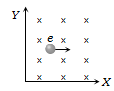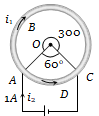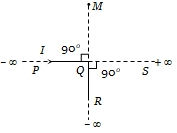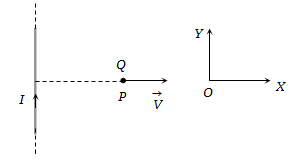A proton and a deutron both having the same kinetic energy, enter perpendicularly into a uniform magnetic field B. For motion of proton and deutron on circular path of radius ${R}_{p}$and ${R}_{d}$ respectively, the correct statement is

(a) ${R}_{d}=\sqrt{2}{R}_{p}$            (b) ${R}_{p}={R}_{p}/\sqrt{2}$

(c) ${R}_{d}={R}_{p}$                  (d) ${R}_{d}=2{R}_{p}$

Concept Questions :-

Lorentz force
High Yielding Test Series + Question Bank - NEET 2020

Difficulty Level:

Two straight long conductors AOB and COD are perpendicular to each other and carry currents ${\mathrm{i}}_{1}$ and ${\mathrm{i}}_{2}$ . The magnitude of the magnetic induction at a point P at a distance a from the point O in a direction perpendicular to the plane ACBD is:

1. $\frac{{\mathrm{\mu }}_{0}}{2\mathrm{\pi a}}\left({\mathrm{i}}_{1}+{\mathrm{i}}_{2}\right)$                    2. $\frac{{\mathrm{\mu }}_{0}}{2\mathrm{\pi a}}\left({\mathrm{i}}_{1}-{\mathrm{i}}_{2}\right)$

3. $\frac{{\mathrm{\mu }}_{0}}{2\mathrm{\pi a}}{\left({\mathrm{i}}_{1}^{2}+{\mathrm{i}}_{2}^{2}\right)}^{1/2}$                4. $\frac{{\mathrm{\mu }}_{0}}{2\mathrm{\pi a}}\frac{{\mathrm{i}}_{1}{\mathrm{i}}_{2}}{\left({\mathrm{i}}_{1}+{\mathrm{i}}_{2}\right)}$

Concept Questions :-

Magnetic field due to various cases
High Yielding Test Series + Question Bank - NEET 2020

Difficulty Level:

The charge on a particle Y is double the charge on particle X. These two particles X and Y after being accelerated through the same potential difference enter a region of the uniform magnetic field and describe circular paths of radii ${R}_{1}$ and ${R}_{2}$ respectively. The ratio of the mass of X to that of Y is:

(a) ${\left(\frac{2{R}_{1}}{{R}_{2}}\right)}^{2}$                      (b) ${\left(\frac{{R}_{1}}{2{R}_{2}}\right)}^{2}$

(c)  $\frac{{R}_{1}^{2}}{2{R}_{2}^{2}}$                           (d) $\frac{2{R}_{1}}{{R}_{2}}$

Concept Questions :-

Lorentz force

Difficulty Level:

In the given figure, the electron enters into the magnetic field. It deflects in ...... direction(a) + ve X direction

(b) – ve X direction

(c) + ve Y direction

(d) – ve Y direction

Concept Questions :-

Lorentz force
High Yielding Test Series + Question Bank - NEET 2020

Difficulty Level:

A cell is connected between the points A and C of a circular conductor ABCD of centre O with angle AOC = $60°$ . If ${\mathrm{B}}_{1}$ and ${\mathrm{B}}_{2}$ are the magnitudes of the magnetic fields at O due to the currents in ABC and ADC respectively, the ratio $\frac{{\mathrm{B}}_{1}}{{\mathrm{B}}_{2}}$ is:1. 0.2

2. 6

3. 1

4. 5

Concept Questions :-

Magnetic field due to various cases
High Yielding Test Series + Question Bank - NEET 2020

Difficulty Level:

An electron, a proton, a deuteron and an alpha particle, each having the same speed are in a region of constant magnetic field perpendicular to the direction of the velocities of the particles. The radius of the circular orbits of these particles are respectively ${R}_{e}$, ${R}_{p}$${R}_{d}$ and ${R}_{\alpha }$. It follows that

(a) ${R}_{e}={R}_{p}$                (b) ${R}_{p}={R}_{d}$

(c) ${R}_{d}={R}_{\alpha }$                 (d) ${R}_{p}={R}_{\alpha }$

Concept Questions :-

Lorentz force
High Yielding Test Series + Question Bank - NEET 2020

Difficulty Level:

An infinitely long conductor PQR is bent to form a right angle as shown. A current I flows through PQR. The magnetic field due to this current at the point M is H1. Now another infinitely long straight conductor QS is connected at Q so that the current is I/2 in QR as well as in QS, The current in PQ remaining unchanged. The magnetic field at M is now ${H}_{\mathit{2}}$The ratio ${H}_{\mathit{1}}\mathit{/}{H}_{\mathit{2}}$ is given by(a) $\frac{1}{2}$

(b)  1

(c)  $\frac{2}{3}$

(d)  2

Concept Questions :-

Magnetic field due to various cases
High Yielding Test Series + Question Bank - NEET 2020

Difficulty Level:

A very long straight wire carries a current I. At the instant when a charge $+\underset{~}{O}$ at point P has velocity $\stackrel{\to }{v}$ , as shown, the force on the charge is:1. Opposite to OX                            2. Along OX

3. Opposite to OY                             4. Along OY

Concept Questions :-

Lorentz force
High Yielding Test Series + Question Bank - NEET 2020

Difficulty Level:

An electric field of 1500 V / m and a magnetic field of 0.40 weber / $mete{r}^{2}$ act on a moving electron. The minimum uniform speed along a straight line the electron could have is

(a) $1.6×{10}^{15}m/s$                          (b) $6×{10}^{-16}m/s$

(c) $3.75×{10}^{3}m/s$                          (d) $3.75×{10}^{2}m/s$

Concept Questions :-

Lorentz force
High Yielding Test Series + Question Bank - NEET 2020

Difficulty Level:

A coil having N turns is wound tightly in the form of a spiral with inner and outer radii a and b respectively. When a current I passes through the coil, the magnetic field at the centre is:

1. $\frac{{\mathrm{\mu }}_{0}\mathrm{NI}}{\mathrm{b}}$                                  2. $\frac{2{\mathrm{\mu }}_{0}\mathrm{NI}}{\mathrm{a}}$

3. $\frac{{\mathrm{\mu }}_{0}\mathrm{NI}}{2\left(\mathrm{b}-\mathrm{a}\right)}\mathrm{ln}\frac{\mathrm{b}}{\mathrm{a}}$                         4. $\frac{{\mathrm{\mu }}_{0}{\mathrm{I}}^{\mathrm{N}}}{2\left(\mathrm{b}-\mathrm{a}\right)}\mathrm{ln}\frac{\mathrm{b}}{\mathrm{a}}$

Concept Questions :-

Magnetic field due to various cases